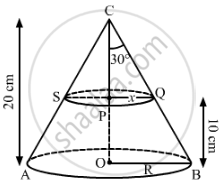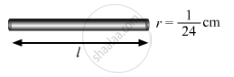# A solid metallic right circular cone 20 cm high and whose vertical angle is 60°, is cut into two parts at the middle of its height by a plane parallel to its base. If the frustum so obtained be drawn into a wire of diameter 1/12 cm, find the length of the wire. - Mathematics

A solid metallic right circular cone 20 cm high and whose vertical angle is 60°, is cut into two parts at the middle of its height by a plane parallel to its base. If the frustum so obtained be drawn into a wire of diameter 1/12 cm, find the length of the wire.

#### SolutionLet ACB be the cone whose vertical angle ∠ACB = 60°. Let R and x be the radii of the lower and upper end of the frustum.
Here, height of the cone, OC = 20 cm = H
Height CP = h = 10 cm
Let us consider P as the mid-point of OC.
After cutting the cone into two parts through P,

OP = 20/2=10 cm
Also, ∠ACO and ∠OCB = (1/2)×60°=30°
After cutting cone CQS from cone CBA, the remaining solid obtained is a frustum.

Now, in triangle CPQ:

tan30^@=x/10

⇒1/sqrt3=x/10

⇒x=10/sqrt3cm

In triangle COB:

tan30^@=R/"CO"

⇒1/sqrt3=R/20

⇒R=20/sqrt3 cm

Volume of the frustum, V=1/3π(R2H − x2h)

⇒V=1/3π((20/sqrt3)^2⋅20−(10/sqrt3)^2⋅10)

=1/3π(8000/3−1000/3)

= 1/3π(7000/3)

=1/9π×7000

=7000/9π

The volumes of the frustum and the wire formed are equal.

πxx(1/24)^2xxl=7000/9π [Volume of wire =πr2h]

⇒l=7000/9xx24xx24

⇒l=448000 cm=4480 m

Hence, the length of the wire is 4480 m.

Concept: Concept of Surface Area, Volume, and Capacity
Is there an error in this question or solution?
2013-2014 (March) Delhi Set 1
Share